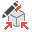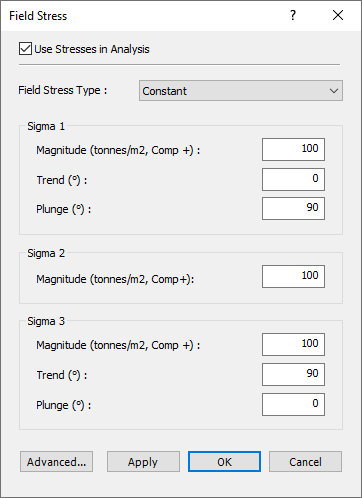RSWebinar: Designing Cost-Effective Ground Improvement Solutions with Settle3 | June 7th, 2023 Register Now

# Field Stress Overview

The default UnWedge analysis is based upon the assumption that the wedges are subjected to gravitational loading only. This means that the stresses on the joint planes are primarily due to the self-weight of each wedge (as well as support forces, water pressure, seismic forces, etc.). The actual stress field in the rock mass surrounding the excavation is not taken into account.

While this assumption leads to some inaccuracy in the analysis, the error is generally conservative, leading to a lower Factor of Safety. However, you may include the effect of in-situ stress on the wedges, with the Field Stress option.

• In the UnWedge implementation, the inclusion of Field Stress can only increase the Factor of Safety, it cannot lower the Factor of Safety.
• Field Stress only applies to Perimeter Wedges, and cannot be applied to End Wedges (see below for more info).

The Field Stress option works generally as follows:

1. Select the Field Stressoption from the Analysis menu.
2. You may see a Warning dialog, with some information about using the Field Stress option. If you see this dialog, select OK to proceed to the Field Stress dialog.
3. In the Field Stress dialog, select the Use Stresses in Analysis checkbox.4. Select the Field Stress Type ( Constant or Gravitational) and enter the required parameters. See the Constant Field Stress and Gravitational Field Stress topics for more information.
5. You may also configure advanced settings relating to the stress analysis, by selecting the Advanced button in the Field Stress dialog. See the Advanced Stress Settings topic for more information.
6. Select Apply or OK to compute the stress analysis and view the effects on the UnWedge analysis results.

## How Does Stress Analysis Work?

When you use the Field Stress option (as described above), the stress analysis is carried out and used in the UnWedge analysis as follows:

1. The first thing that happens, is that UnWedge will carry out a stress analysis around your excavation. The stress analysis uses the boundary element method, which assumes linear elastic behaviour (i.e., plasticity and localized failure is not accounted for in this type of analysis).
2. The boundary element stress analysis used in UnWedge is based on the analysis engine used in the Rocscience program EX3. Click here for more information about EX3.
3. The boundary element stress analysis in UnWedge uses the Complete Plane Strain formulation. This means that the principal stress orientations do not have to be aligned with the excavation axis. Although the excavation model is 2-dimensional, the defined Field Stress and the stress analysis results are actually 3-dimensional.
4. The stress analysis determines the stress distribution around the Opening Section assuming an infinitely long excavation in the axis direction. The stress analysis DOES NOT analyze the stress distribution around the ends of the excavation.
5. Therefore, the results of using Field Stress can only be applied to the Perimeter Wedges and does not apply to the End Wedges.
6. UnWedge can then determine the actual distribution of stress on each wedge face (Perimeter Wedges only) and include this stress in the stability calculations.

## Warning about Using Field Stress

As mentioned in the Warning dialog (see above), the inclusion of Field Stress in the UnWedge analysis can only increase the Factor of Safety of a wedge. That is, the Field Stress can only act to "clamp" the wedge in place. The stress cannot lower the Factor of Safety, or "push" a wedge out - these mechanisms are not modelled in the UnWedge analysis, even though they may in reality occur.

If the stress around the excavation has caused some wedge movement to occur, then this implies that the stress is no longer being transferred through the wedge. In this case, the default UnWedge analysis applies (i.e., gravitational loading of the wedge due to self-weight), and you should not use the Field Stress option. If long-term behaviour is being considered, then Field Stress should not be used, so that the analysis and design of support is conservative.

## Speed of Calculations When Using Field Stress

If you use the Field Stress option, this can noticeably affect the speed of the UnWedge calculations. This is because the boundary element stress analysis must be carried out first, before the limit equilibrium calculations. If the tunnel axis orientation is changed, the stress analysis must be re-computed. If joint orientations are changed, the stresses must be re-computed on each wedge face.

You may not notice any difference in speed for a simple analysis of a single tunnel orientation. However, if you are using the Tunnel Axis Plot option, the plot generation may take some time if you are using Field Stress because the entire boundary element analysis has to be re-computed for each tunnel orientation.

## Probabilistic Field Stress

Variability can also be added to the Field Stress properties. See the Probabilistic Field Stress option for more information.